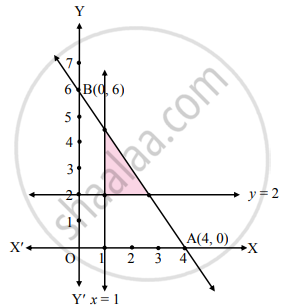# Solve the following system of inequalities graphically.3x + 2y ≤ 12, x ≥ 1, y ≥ 2 - Mathematics and Statistics

Graph

Solve the following system of inequalities graphically.
3x + 2y ≤ 12, x ≥ 1, y ≥ 2

#### Solution

To find graphical solution, construct the table as follows:

 Inequation Equation Double Intercept form Points (x, y) Region 3x + 2y ≤ 12 3x + 2y = 12 "x"/4 + "y"/6 = 1 A (4, 0),B (0, 6) 3(0) + 2(0) ≤ 12∴ 0 ≤ 12∴ origin side x ≥ 1 x = 1 – – 0 ≱  1∴ R.H.S. of line x = 1 y ≥ 2 y = 2 – – 0 ≱  2∴ above liney = 2The shaded portion represents the graphical solution.

Concept: Solution of System of Linear Inequalities in Two Variables
Is there an error in this question or solution?

#### APPEARS IN

Balbharati Mathematics and Statistics 2 (Commerce) 11th Standard HSC Maharashtra State Board
Chapter 8 Linear Inequations
Miscellaneous Exercise 8 | Q 2 | Page 122